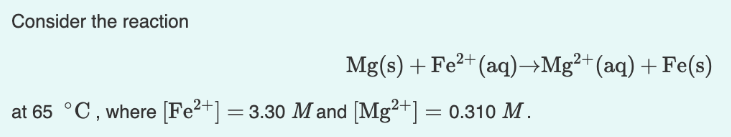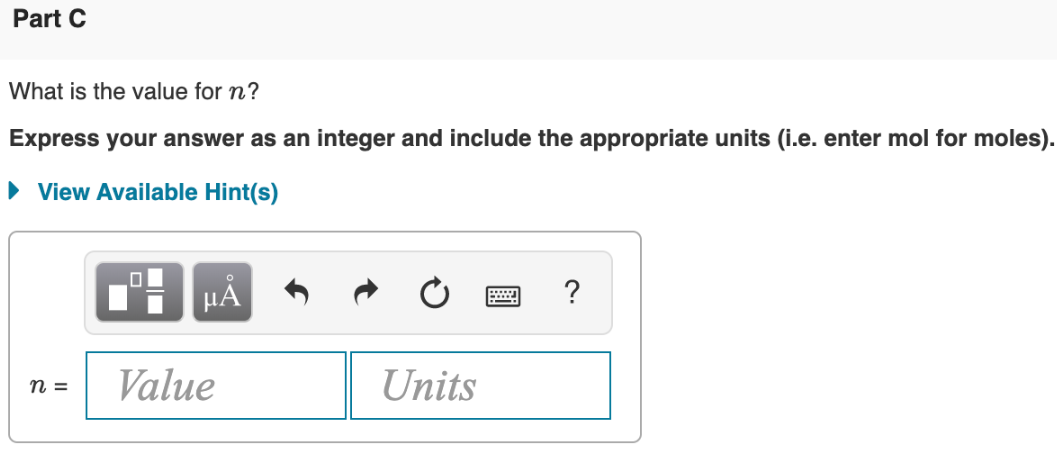# Consider the reaction Mg(s) + Fe^2+(aq) → Mg^2+(aq) + Fe(s) at 65 °C, where [Fe^2+] = 3.30 M and [Mg^2+] = 0.310 M. What is the value for n? Express your answer as an integer and include the appropriate units (i.e. enter mol for moles).